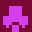# bit-arrays

#### Accessor words for bit arrays

```\ FORTH BIT Arrays , by Bob Dickow dickow@turbonet.com
\ version 3.0.2 Mar 6, 2018
\ Notes:
\ endian agnostic, address unit agnostic (untested)
\ Lexicon: BIT@ BIT! BOOL@ BOOL!

\ N.B. Buffers for a bit array should be a minimum of 1 CELL in size

DECIMAL

CELL 8 * 1-  VALUE ShiftV

\ a factoring, internal use by BIT@,  BOOL@

: _BIT@    ( addr n-index -- n )
CELL 8 * /MOD 	( addr n n )
CELLS        ( addr n n )
ROT  +       ( n addr )
@ SWAP       ( nval n )
1+ 1 ShiftV ROT  - LSHIFT
AND
;

\ given a memory buffer address addr and index n representing at bit-wise
\ index, return the value of the bit at that index as 1 | 0.

: BIT@   ( addr n-index -- n )
_BIT@  ( n )
0<>
ABS
;

\ : NOOP ( -- ) ;
\ conditionally compile NOOP do-nothing word if it isn't in the implementation:
." NOOP" FORTH-WORDLIST SEARCH-WORDLIST 0= [IF]  : NOOP ; [ELSE] DROP [THEN]

\ store a value TRUE|FALSE or 1|0, given the value (TRUE|FALSE or 1|0), an address, and a bit-wise index
\ (synonym for BOOL! below)

: BIT!   ( n-value addr n-index -- )
ROT ABS              ( addr n n )
IF ['] NOOP [']  OR  ELSE ['] INVERT ['] AND    THEN >R >R  \ set up masking OP
CELL 8 * /MOD 		   ( addr n n )
CELLS                ( addr n n )
1+ 1 ShiftV ROT  - LSHIFT
R> EXECUTE R> EXECUTE    ( addr n )
SWAP !
;

\ given a memory buffer address addr and index n representing at bit-wise
\ offset, return or store the value of the bit as TRUE|FALSE values.

: BOOL@   ( addr n-index -- flg )
_BIT@  	  ( n )
0<>       ( n )
;

\  Synonym for BIT!
\  store a value TRUE|FALSE ( or 1|0 ), given the value n, a base address of the array, and a bit-wise index into it.

: BOOL!   ( n addr n-index --  )
BIT!
;

```

## by Budsy